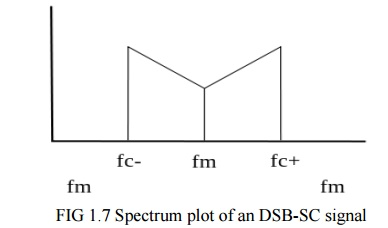Home | | Communication Theory | Double-sideband suppressed-carrier transmission (DSB-SC)

# Double-sideband suppressed-carrier transmission (DSB-SC)

Double-sideband suppressed-carrier transmission (DSB-SC) is transmission in which frequencies produced by amplitude modulation (AM) are symmetrically spaced above and below the carrier frequency and the carrier level is reduced to the lowest practical level, ideally being completely suppressed.

DSB-SC:

Double-sideband suppressed-carrier transmission (DSB-SC) is transmission in which frequencies produced by amplitude modulation (AM) are symmetrically spaced above and below the carrier frequency and the carrier level is reduced to the lowest practical level, ideally being completely suppressed.

ü   Spectrum:

DSB-SC is basically an amplitude modulation wave without the carrier, therefore reducing power waste, giving it a 50% efficiency. This is an increase compared to normal AM transmission (DSB), which has a maximum efficiency of 33.333%, since 2/3 of the power is in the carrier which carries no intelligence, and each sideband carries the same information. Single Side Band (SSB) Suppressed Carrier is 100% efficient.ü   Generation:

DSB-SC is generated by a mixer. This consists of a message signal multiplied by a carrier signal. The mathematical representation of this process is shown below, where the product-to-sum trigonometric identity is used.ü   Demodulation:

Demodulation is done by multiplying the DSB-SC signal with the carrier signal just like the modulation process. This resultant signal is then passed through a low pass filter to produce a scaled version of original message signal. DSB-SC can be demodulated if modulation index is less than unity.The equation above shows that by multiplying the modulated signal by the carrier signal, the result is a scaled version of the original message signal plus a second term. Since , ωc>>ωm wthis second term is much higher in frequency than the original message.

Once this signal passes through a low pass filter, the higher frequency component is removed, leaving just the original message.

ü   Distortion and Attentuation:

For demodulation, the demodulation oscillator's frequency and phase must be exactly the same as modulation oscillator's, otherwise, distortion and/or attenuation will occur.

To see this effect, take the following conditions:Study Material, Lecturing Notes, Assignment, Reference, Wiki description explanation, brief detail
Communication Theory : Amplitude Modulation : Double-sideband suppressed-carrier transmission (DSB-SC) |